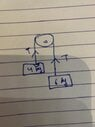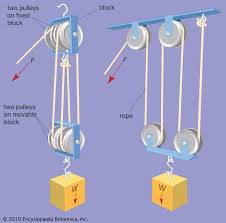# Atwood machine -- Question about the pulley and weights

Gold Member
I want to ask in this machine when there are two masses hanging down then the tension T is directed upwards along the rope. Is it the force applied by the rope on the mass? Is it the force applied by the pulley? When the anyone mass of the machine moves downwards is it because the force of gravity >tension T ?

#### Attachments

•image.jpg
21.3 KB · Views: 34
Last edited:

Mentor
Assume an ideal massless unstrechable rope and an ideal frictionless pulley. Assume that the pulley is fastened to the ceiling or otherwise secured because you did say “Atwood machine”

The rope applies an upwards force on the mass and gravity applies a downwards force. Depending on which is greater the mass will accelerate upwards or downward.

The pulley is applying a force on the rope, not the masses.

Mentor
A rope under tension exerts a force at each end. So the rope pulls up on the mass and down on the pulley. And if the downward force of gravity is greater than the tension pulling up on a mass, that mass will accelerate downward. (And vice versa.)

Just apply Newton's 2nd law to each mass.

(Oops... Nug beat me to it.)

•rudransh verma
Homework Helper
Gold Member
The only function of the pulley is to change direction of the force or tension in the rope or string keeping those two bodies linked together and behaving as one.
It can change direction several times.http://hyperphysics.phy-astr.gsu.edu/hbase/atwd.html

When the system moves, the force of gravity could be equal to (mass moves at constant velocity) or greater than (mass accelerates) the string tension T.

Last edited:
Homework Helper
then the tension T is directed upwards along the rope
Strictly speaking, tension is neither "upwards" nor "downwards". It is vertical. It is a condition in the rope. A third law force pair between each and every incremental section of rope and the next. A force pair does not point in one direction or the other. It points in both.

At the ends of the cord, the tension can act in only one of those two directions. It pulls upward on the connected object at the bottom and downward on the connected object at the top.

•rudransh verma
Gold Member
The pulley is applying a force on the rope, not the masses.
The gravity is applying the force on mass. Rope is applying force on mass in upward direction. Similarly, rope is applying force on pulley in downward direction and pulley is to the rope in upward direction. Got it!
Thanks @Doc Al

Gold Member
The only function of the pulley is to change direction of the force or tension in the rope or string keeping those two bodies linked together and behaving as one.
Is this diagram correct. Is there a T in downward direction too?

#### Attachments

Last edited:
Homework Helper
Is this diagram correct. Is there a T in downward direction too?
You have ##T_I## written twice on that drawing. What are either of them supposed to be labelling?

Gold Member
You have ##T_I## written twice on that drawing. What are either of them supposed to be labelling?
Tension?

Homework Helper
Tension?
Tension as a condition in the two segments of cord? That would be fine.

Tension as a supporting force applied to the weights? That would also be fine. Though it could then appear four times - once on each weight and twice on the pulley.

Just the word "tension" by itself is unenlightening. Use your words and say what you mean.

Homework Helper
Gold Member
Is this diagram correct. Is there a T in downward direction too?
What are those green arrows representing?

Gold Member
Just the word "tension" by itself is unenlightening. Use your words and say what you mean.
So there are four Tensions in this machine, all T. Can be more in complex machines!
What are those green arrows representing?
I don't know